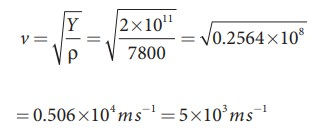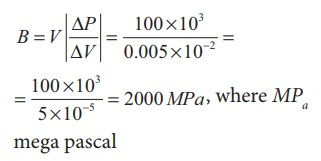Home | | Physics 11th std | Solved Example Problems for Velocity of Waves in Different Media

# Solved Example Problems for Velocity of Waves in Different Media

Physics : Waves - Solved Example Problems for Velocity of Waves in Different Media

## Velocity of transverse waves in a stretched string

### EXAMPLE 11.6

Calculate the velocity of the travelling pulse as shown in the figure below. The linear mass density of pulse is 0.25 kg m-1. Further, compute the time taken by the travelling pulse to cover a distance of 30 cm on the string.### Solution

The tension in the string is T = m g = 1.2 × 9.8 = 11.76 N

The mass per unit length is μ = 0.25 kg m-1. Therefore, velocity of the wave pulse isThe time taken by the pulse to cover the distance of 30 cm iswhere

ms = milli second.

## Velocity of longitudinal waves in an elastic medium

### EXAMPLE 11.7

Calculate the speed of sound in a steel rod whose Young’s modulus Y = 2 × 1011 N m-2 and ρ = 7800 kg m-3.

### SolutionTherefore, longitudinal waves travel faster in a solid than in a liquid or a gas. Now you may understand why a shepherd checks before crossing railway track by keeping his ears on the rails to safegaurd his cattle.

### EXAMPLE 11.8

An increase in pressure of 100 kPa causes a certain volume of water to decrease by 0.005% of its original volume.

(a) Calculate the bulk modulus of water?.

(b) Compute the speed of sound (compressional waves) in water?.

### Solution

(a) Bulk modulus(b) Speed of sound in water isTags : Physics , 11th Physics : UNIT 11 : Waves
Study Material, Lecturing Notes, Assignment, Reference, Wiki description explanation, brief detail
11th Physics : UNIT 11 : Waves : Solved Example Problems for Velocity of Waves in Different Media | Physics